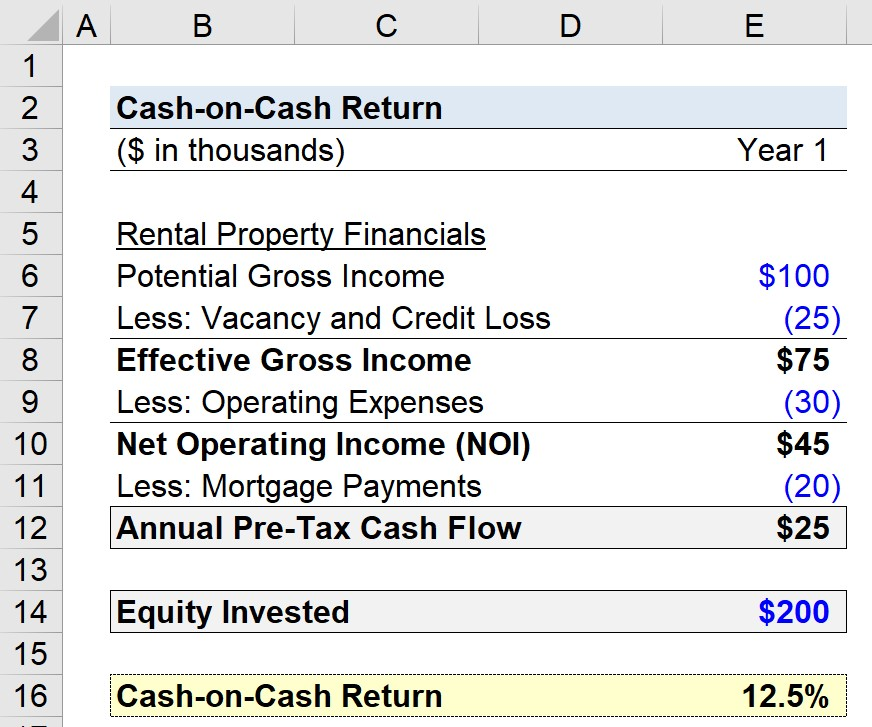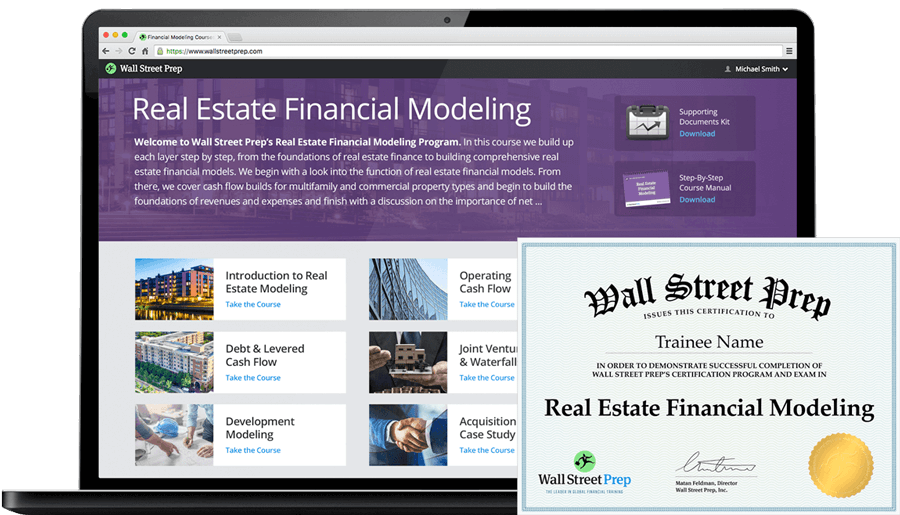Welcome to Wall Street Prep! Use code at checkout for 15% off.# Cash on Cash Return

Guide to Understanding the Cash on Cash Return (Cash Yield)## How to Calculate Cash on Cash Return?

The cash on cash return, or “cash yield”, measures a real estate investor’s annual pre-tax earnings on a property relative to the initial amount spent to purchase the property itself.

The cash on cash return is calculated as the ratio between the annual pre-tax cash flow and invested equity:

1. Annual Pre-Tax Cash Flow → The annual pre-tax cash income generated on the property investment.
2. Invested Equity → The initial equity investment, i.e. the outlay of cash on the date of purchase.

In practice, the cash-on-cash return metric estimates the annual yield received by an investor on a specific property relative to the amount paid in the corresponding year, e.g. the mortgage payments.

Since the cash yield factors in costs like mortgage payments, the effects of financing costs are part of the implied return.## Cash on Cash Return Formula

The formula for calculating the cash-on-cash return involves taking the annual pre-tax cash flow and dividing it by the initial cash investment, i.e. the equity contribution.

Cash on Cash Return (%) = Annual Pre-Tax Cash Flow ÷ Invested Equity

While the numerator is pre-tax, the metric is calculated post-financing, so the annual cash flow is a “levered” metric.

The cash yield is expressed as a percentage, which makes comparisons across different property investment opportunities easier.

However, the context in which the investment was completed, such as the location, date, and real estate market conditions, must all be taken into account.

Common examples of expenses that are factored into the cash flow metric are the following:

• Property Taxes
• Maintenance Fees
• Renovation Fees
• Closing Fees

## Cash on Cash Return vs. Cap Rate: What is the Difference?

The difference between the cash-on-cash return and the cap rate is as follows.

• Cash on Cash Return → The cash on cash return, or “cash yield”, measures the annual pre-tax cash flow received per dollar of equity invested and determined on a post-financing basis. The numerator is unaffected by taxes; however, is calculated after deducting financing costs, such as annual mortgage payments and interest.
• Cap Rate (%) → In contrast, the cap rate, or “capitalization rate”, is a fundamental metric in real estate investing that measures the expected rate of return to be received on an investment property. Unlike the cash-on-cash return, the cap rate neglects the effects of financing, since the numerator is net operating income (NOI), an unlevered profit metric unaffected by discretionary financing decisions, i.e. capital structure neutral. For instance, the cap rate is not inclusive of financing costs, such as mortgage costs, contrary to the cash-on-cash return.

The cap rate is calculated by dividing the annual net operating income (NOI) by the market value of the property, while the cash on cash return is determined by dividing the levered pre-tax cash flow by the equity contribution.

Cap Rate (%) = Net Operating Income (NOI) ÷ Property Value

In short, the denominator in the cap rate formula is the market value of the property – i.e. the fair value of the property at present – while the denominator in the cash-on-cash return formula is the equity contribution by the investor.

## Cash on Cash Return vs. ROI: What is the Difference?

By now, we understand that the cash on cash return (or cash yield) measures the annual pre-tax cash flow compared to the initial amount of cash invested.

In contrast, the return on investment (ROI) calculates the yield across the entire holding period, whereas the cash yield usually covers the current period, i.e. only one year.

• Return on Investment (ROI) → Entire Holding Period
• Cash on Cash Return (CoC) → Current Period, i.e. One-Year (or Twelve Months)

The cash-on-cash return (CoC) can be considered the return over a short time frame, whereas the return on investment (ROI) is a cumulative returns metric.

Unlike the return on investment (ROI), the cash yield can increase (or decrease) periodically due to fluctuations in rental income, expenses, and other related external factors.

Another differentiation between the two metrics appears in the topic of debt service. The cash flow metric in the CoC return calculation is only reduced by the debt service in the current period.

However, the return on investment (ROI) metric considers the entirety of the debt obligations related to the property investment.

Hence, the cash yield metric is most applicable for real estate property investments funded by debt capital.

If debt financing was used as part of the transaction – which is usually the case in the commercial real estate market – then the actual cash return on the investment diverges from the return on investment (ROI).

## What is a Good Cash on Cash Return?

There is not necessarily a universally “good” cash yield return that all real estate investors target, considering each investor sets their own return (and risk) targets.

Generally speaking, the real estate market consensus is that a forecasted cash-on-cash return between 8% and 12% is considered a worthwhile investment.

Market conditions are another factor that must be considered, as well as the type of properties (and geographical location) of the investments made.

For the reasons above, it is difficult to quantify a specific, universal return to target, as it is subjective and affected by numerous variables.

## Cash-on-Cash Return Calculator

We’ll now move on to a modeling exercise, which you can access by filling out the form below.#### Get the Excel Template!Submitting...

## 1. Commercial Real Estate Property Assumptions

Suppose a commercial real estate investor purchased a rental property with a potential gross income of \$100,000. But because of vacancies and credit losses, there is a deduction of \$25,000.

The effective gross income (EGI) is thus \$75,000.

• Potential Gross Income = \$100,000
• Vacancy and Credit Loss = \$25,000
• Effective Gross Income (EGI) = \$100,000 – \$25,000 = \$75,000

## 2. Net Operating Income Calculation (NOI)

In the next step, we’ll assume the operating expenses related to the property amounted to \$30,000, so the net operating income (NOI) is \$45,000.

• Operating Expenses = \$30,000
• Net Operating Income (NOI) = \$75,000 – \$30,000 = \$45,000

## 3. Mortgage Payments and Pre-Tax Income Calculation

We’ll now subtract the debt-related payments for the current year – i.e. the mortgage payments, such as interest and principal repayment – which we’ll assume to be \$20,000.

• Mortgage Payments = \$20,000

By subtracting the rental property’s mortgage payments from its net operating income (NOI), we calculate the annual pre-tax cash flow as \$25,000.

• Annual Pre-Tax Cash Flow = \$45,000 – \$20,000 = \$25,000

## 4. Cash-on-Cash Return Calculation Example

In the final section of our exercise, the only remaining assumption needed is the initial amount of equity invested, or \$200,000.

• Equity Invested = \$200,000

After dividing our annual pre-tax cash flow by the equity invested, the implied cash-on-cash return comes out to 12.5%.

• Cash-on-Cash Return (%) = \$25,000 ÷ \$200,000 = 12.5%20+ Hours of Online Video Training

### Master Real Estate Financial Modeling

This program breaks down everything you need to build and interpret real estate finance models. Used at the world's leading real estate private equity firms and academic institutions.

Inline Feedbacks